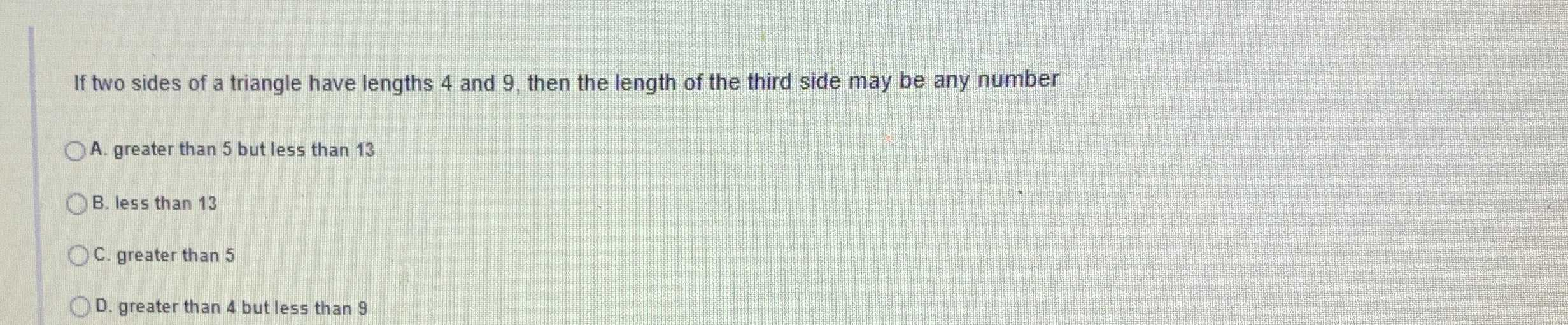### Still have math questions?

Trigonometry
QuestionIf two sides of a triangle have lengths $$4$$ and $$9$$ , then the length of the third side may be any number

A. greater than $$5$$ but less than $$13$$

B. greater than $$5$$ but less than $$13$$

C. greater than $$5$$

D. greater than $$4$$ but less than $$9$$